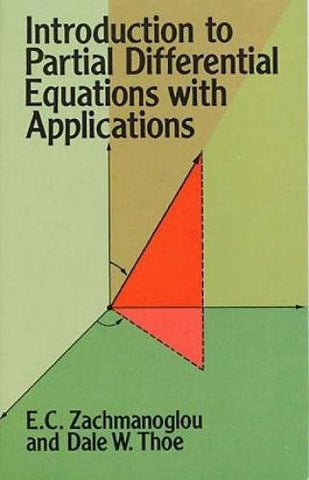# Introduction to Partial Differential Equations with Applications (Dover Books on Mathematics)

• \$29.33
• Save \$3.42
• Binding: Paperback
• Author: E. C. Zachmanoglou;Dale W. Thoe;Mathematics
• Publish Date: 1987-02-01

Attention : For textbook, access codes and supplements are not guaranteed with used items.

This book has been widely acclaimed for its clear, cogent presentation of the theory of partial differential equations, and the incisive application of its principal topics to commonly encountered problems in the physical sciences and engineering. It was developed and tested at Purdue University over a period of five years in classes for advanced undergraduate and beginning graduate students in mathematics, engineering and the physical sciences.
The book begins with a short review of calculus and ordinary differential equations, then moves on to explore integral curves and surfaces of vector fields, quasi-linear and linear equations of first order, series solutions and the Cauchy Kovalevsky theorem. It then delves into linear partial differential equations, examines the Laplace, wave and heat equations, and concludes with a brief treatment of hyperbolic systems of equations.
Among the most important features of the text are the challenging problems at the end of each section which require a wide variety of responses from students, from providing details of the derivation of an item presented to solving specific problems associated with partial differential equations. Requiring only a modest mathematical background, the text will be indispensable to those who need to use partial differential equations in solving physical problems. It will provide as well the mathematical fundamentals for those who intend to pursue the study of more advanced topics, including modern theory.